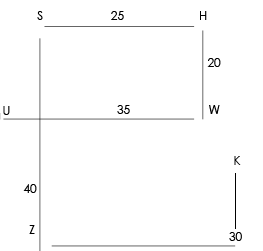Breaking News
Home / Placement Papers / Cummins / Cummins Reasoning Questions

# Cummins Reasoning Questions

## Reasoning QuestionsStudy the following information carefully and answer the questions given below:

Seven persons -A, B, C, D, K, L and N-are seated in ascending order of their salaries. N earns more than L and D. N earns more than A but he does not earn the highest. A earns more than L The person who earns the second highest receives a salary of Rs. 35,000 while the third lowest earner receives Rs. 23,000. K earn less than L but more than D. C earns Rs. 18,000.D is not the lowest earner.

1.Who among the following earn(s) more than Rs. 23,000 but less than Rs. 35,000?

A.Only A
B.Only L
C.A and L
D.K and L
E.A and N

Answer & Explanation

Answer: Option C.

B –
N – Rs 35000
A –
L –
K – Rs 23000
D –
C – Rs 18000
A and L more than Rs. 23,000 but less than Rs. 35,000.

2.Who among the following may earn Rs. 21,000?

A.K
B.D
C.L
D.C
E.N

Answer & Explanation

Answer: Option B.

B –
N – Rs 35000
A –
L –
K – Rs 23000
D –
C – Rs 18000
D earns Rs. 21,000

3.Who among the following earns Rs. 35,000?

A.A
B.L
C.B
D.K
E.N

Answer & Explanation

Answer: Option E.

B –
N – Rs 35000
A –
L –
K – Rs 23000
D –
C – Rs 18000
N earns Rs 35,000

In these questions, relationships between elements are shown in the statements. These statements are followed by two conclusions. Give answer

A. if only conclusion I follows.
B. if only conclusion II follows.
C. if either conclusion I or conclusion II follows.
D. if neither conclusion I nor conclusion II follows.
E. if both conclusions I and II follow.

4.Statements: G > F = L > J; J > K = H
Conclusions: I. H = G II. G > H

Answer & Explanation

Answer: Option C.

Statements: G > F = L > J; J > K = H
Conclusions: I. H = G II. G < H
G > F = L > J> K = H
From above statement we can conclude that either I or II conclusion follows.

5.Statements: P < R < S < T > U
Conclusions: I. U < R II. T > P

Answer & Explanation

Answer: Option B.

Statements: P < R < S < T > U
Conclusions: I. U < R II. T > P
We can clearly see that conclusion I i:e U < R is false and conclusion II i:e T > P is true.

6.Statements: T > U > V > W; X < Y = W > Z

Conclusions: I. Z > U II. W > T

Answer & Explanation

Answer: Option D.

Statements: T > U > V > W; X < Y = W > Z
Conclusions: I. Z > U II. W > T
T > U > V > W> Z
We can clearly see that conclusion I i:e Z > U and conclusion II i:e W > T are false.

Read the following information and answer the given question.

Point U is 35m to the west of Point W. Point W is 20m to the south of Point H. Point H is 25m to the east of Point S. Shubham is standing at point Z which is 40m to the south of Point S. He starts walking towards east and walks for 30m. He takes a left turn and stops at Point K after walking for 20m.

7.If Shubham walks for 15m towards west from his final position to reach point V, how much distance will he have to cover in order to reach Point U?

A.30m
B.15m
C.35m
D.25m
E.20m

Answer & Explanation

Answer: Option D.Shubham will require moving 25 m in order to reach point U.

8.How far and in which direction is Point W with respect to Point K?

A.10m towards North
B.10m towards West
C.5m towards East
D.5m towards West
E.15m towards East

Answer & Explanation

Answer: Option D.Fill the series

9.4 7 13 25 49 ?

A.118
B.136
C.86
D.97
E.124

Answer & Explanation

Answer: Option D.

The series is : +3,+6,+12,+24,+48

10.50 59 41 68 32 ?

A.77
B.45
C.20
D.85
E.60

Answer & Explanation

Answer: Option A.

It’s a twin series.
The series are : 50, 41, 32 and 59, 68, ?
1st series : -9 and 2nd series : +9

## Test Pattern & Selection Procedure Of Cummins

Test Pattern & Selection Procedure Cummins: Cummins Inc. is one of the major manufacturing industries of …